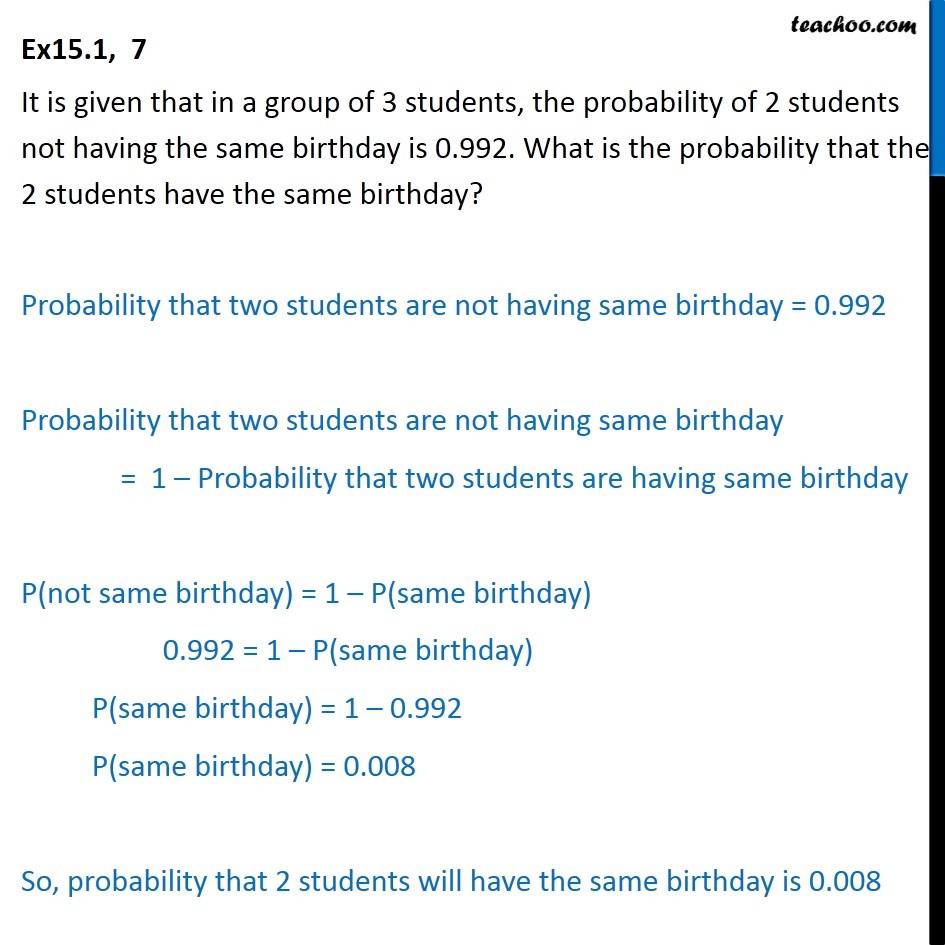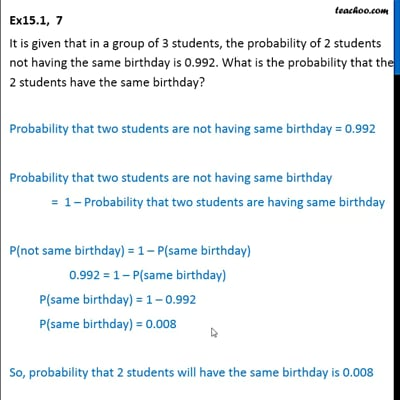Ex 15.1

Chapter 15 Class 10 Probability
Serial order wiseThis video is only available for Teachoo black users

Solve all your doubts with Teachoo Black (new monthly pack available now!)

### Transcript

Ex15.1, 7 It is given that in a group of 3 students, the probability of 2 students not having the same birthday is 0.992. What is the probability that the 2 students have the same birthday? Probability that two students are not having same birthday = 0.992 Probability that two students are not having same birthday = 1 Probability that two students are having same birthday P(not same birthday) = 1 P(same birthday) 0.992 = 1 P(same birthday) P(same birthday) = 1 0.992 P(same birthday) = 0.008 So, probability that 2 students will have the same birthday is 0.008